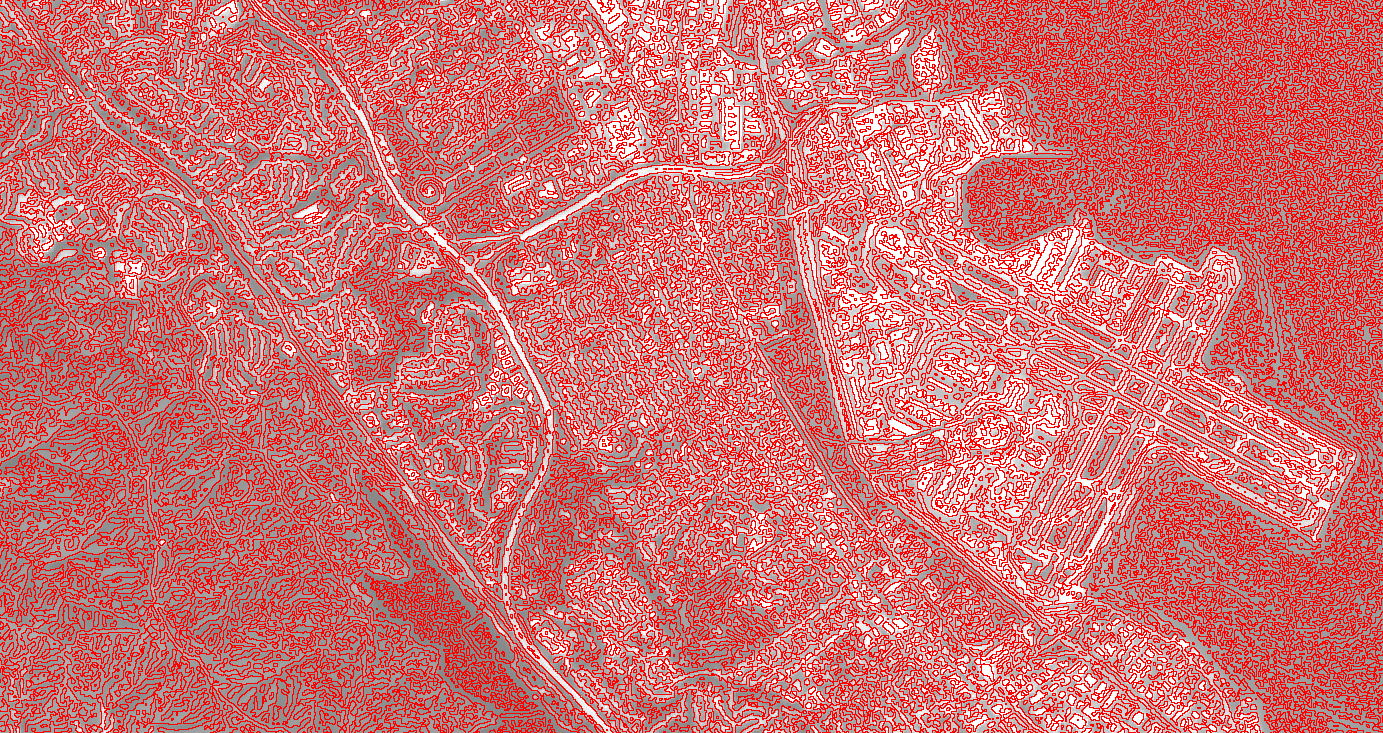# Edge detection

Edge detection is applicable to a wide range of image processing tasks. In addition to the edge detection kernels described in the convolutions section, there are several specialized edge detection algorithms in Earth Engine. The Canny edge detection algorithm (Canny 1986) uses four separate filters to identify the diagonal, vertical, and horizontal edges. The calculation extracts the first derivative value for the horizontal and vertical directions and computes the gradient magnitude. Gradients of smaller magnitude are suppressed. To eliminate high-frequency noise, optionally pre-filter the image with a Gaussian kernel. For example:

### Code Editor (JavaScript)

```// Load a Landsat 8 image, select the panchromatic band.
var image = ee.Image('LANDSAT/LC08/C01/T1/LC08_044034_20140318').select('B8');

// Perform Canny edge detection and display the result.
var canny = ee.Algorithms.CannyEdgeDetector({
image: image, threshold: 10, sigma: 1
});
Map.setCenter(-122.054, 37.7295, 10);
Map.addLayer(canny, {}, 'canny');```

Note that the `threshold` parameter determines the minimum gradient magnitude and the `sigma` parameter is the standard deviation (SD) of a Gaussian pre-filter to remove high-frequency noise. For line extraction from an edge detector, Earth Engine implements the Hough transform (Duda and Hart 1972). Continuing the previous example, extract lines from the Canny detector with:

### Code Editor (JavaScript)

```// Perform Hough transform of the Canny result and display.
var hough = ee.Algorithms.HoughTransform(canny, 256, 600, 100);
Map.addLayer(hough, {}, 'hough');```

Another specialized algorithm in Earth Engine is `zeroCrossing()`. A zero-crossing is defined as any pixel where the right, bottom, or diagonal bottom-right pixel has the opposite sign. If any of these pixels is of opposite sign, the current pixel is set to 1 (zero-crossing); otherwise it's set to zero. To detect edges, the zero-crossings algorithm can be applied to an estimate of the image second derivative. The following demonstrates using `zeroCrossing()` for edge detection:

### Code Editor (JavaScript)

```// Load a Landsat 8 image, select the panchromatic band.
var image = ee.Image('LANDSAT/LC08/C01/T1/LC08_044034_20140318').select('B8');
Map.addLayer(image, {max: 12000});

// Define a "fat" Gaussian kernel.
var fat = ee.Kernel.gaussian({
radius: 3,
sigma: 3,
units: 'pixels',
normalize: true,
magnitude: -1
});

// Define a "skinny" Gaussian kernel.
var skinny = ee.Kernel.gaussian({
radius: 3,
sigma: 1,
units: 'pixels',
normalize: true,
});

// Compute a difference-of-Gaussians (DOG) kernel.
var dog = fat.add(skinny);

// Compute the zero crossings of the second derivative, display.
var zeroXings = image.convolve(dog).zeroCrossing();
Map.setCenter(-122.054, 37.7295, 10);
Map.addLayer(zeroXings.selfMask(), {palette: 'FF0000'}, 'zero crossings');```

The zero-crossings output for an area near the San Francisco, CA airport should look something like Figure 1.Figure 1. Zero-crossings output (red) with the Landsat 8 panchromatic band in the background for an area near the San Francisco, California airport (right).